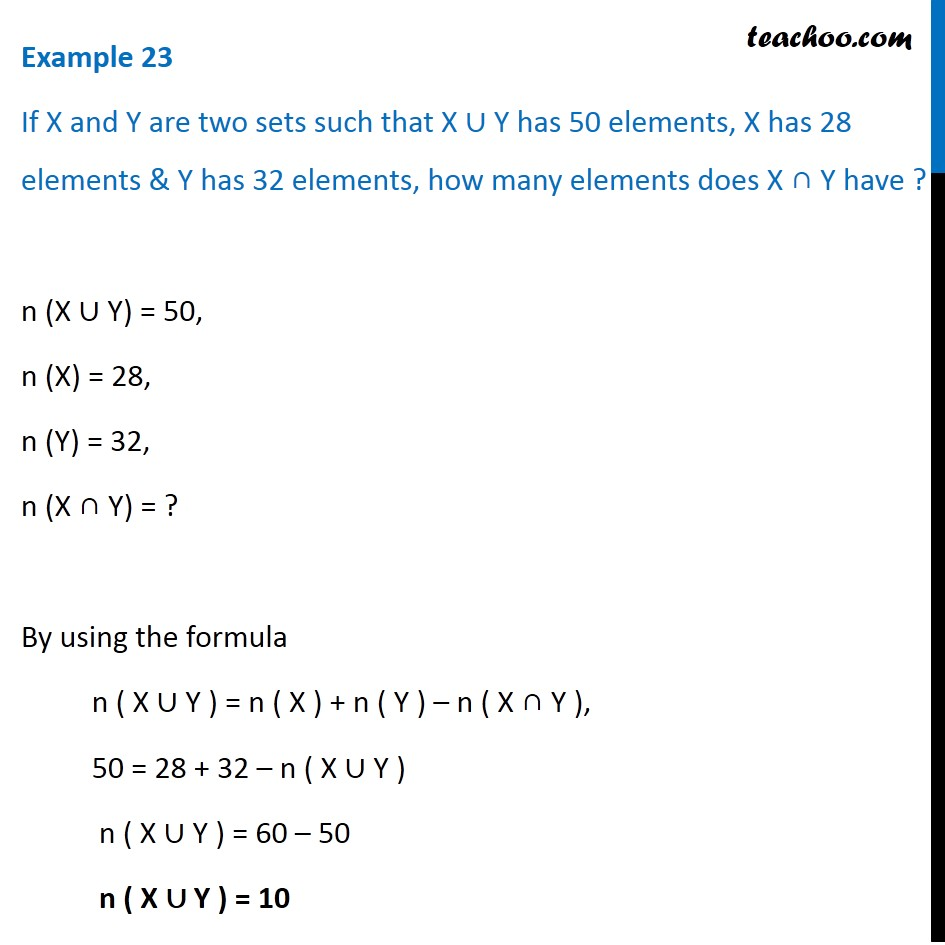Examples

Chapter 1 Class 11 Sets
Serial order wiseLearn in your speed, with individual attention - Teachoo Maths 1-on-1 Class

### Transcript

Question 1 If X and Y are two sets such that X ∪ Y has 50 elements, X has 28 elements & Y has 32 elements, how many elements does X ∩ Y have ? n (X ∪ Y) = 50, n (X) = 28, n (Y) = 32, n (X ∩ Y) = ? By using the formula n ( X ∪ Y ) = n ( X ) + n ( Y ) – n ( X ∩ Y ), 50 = 28 + 32 – n ( X ∪ Y ) n ( X ∪ Y ) = 60 – 50 n ( X ∪ Y ) = 10